# Math in Focus Grade 8 Chapter 3 Lesson 3.2 Answer Key Identifying the Number of Solutions to a Linear Equation

Go through the Math in Focus Grade 8 Workbook Answer Key Chapter 3 Lesson 3.1 Solving Linear Equations with One Variable to finish your assignments.

## Math in Focus Grade 7 Course 3 A Chapter 3 Lesson 3.2 Answer Key Identifying the Number of Solutions to a Linear Equation

### Math in Focus Grade 8 Chapter 3 Lesson 3.2 Guided Practice Answer Key

Tell whether each equation is consistent or inconsistent. Justify your answer by simplifying each equation. Write +, ×, or ÷ for each ?

Question 1.
7(x – 3) – 7x – 21 = 0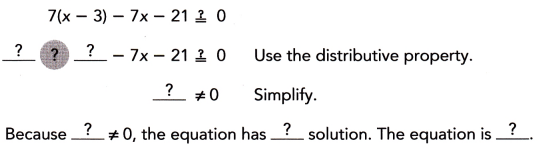The equation has a solution,
the equation is consistent.

Explanation:
Given 7(x-3)-7x – 21 ≠ 0,
7x – 21 – 7x -21 ≠ 0 (using the distributive property),
-42 ≠ 0 because -42 ≠ 0 the equation has a solution,
the equation is consistent.

Question 2.
$$5\left(x+\frac{1}{5}\right)$$ = 5x + 3
Inconsistent,

Explanation: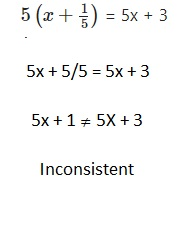Question 3.
x + $$\frac{1}{4}$$ = –$$\frac{1}{4}$$(4x – 1)
The equation has a solution,
the equation is consistent.

Equation: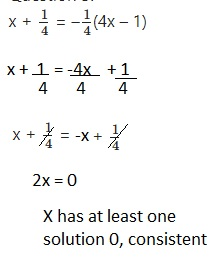Tell whether each equation is an identity. Justify your answer by simplifying each equation.
Write +, -, ×, or ÷ for each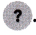Write = or ≠ for each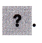Question 4.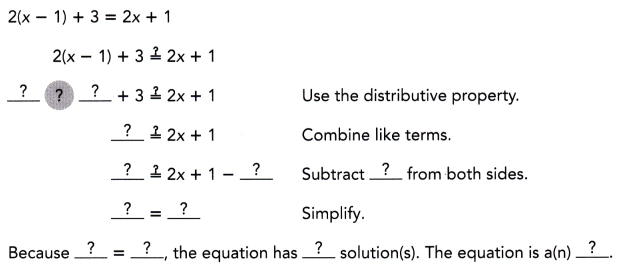Left side is equal to right side,
Equation has an identity,

Explanation: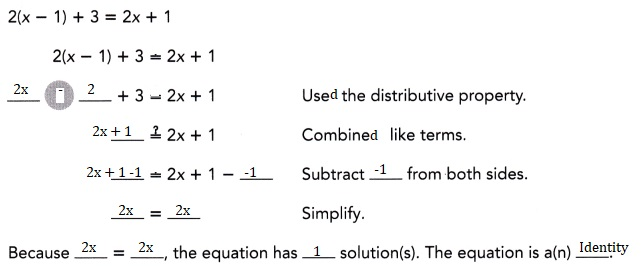Question 5.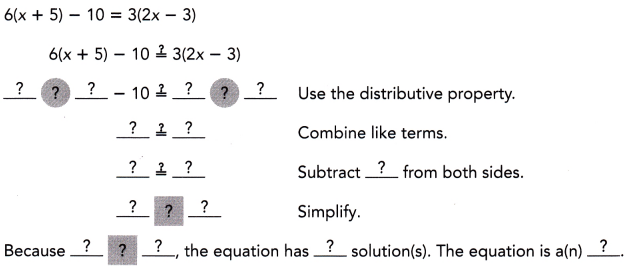Left side is not equal to right side,
Equation has no identity,

Explanation: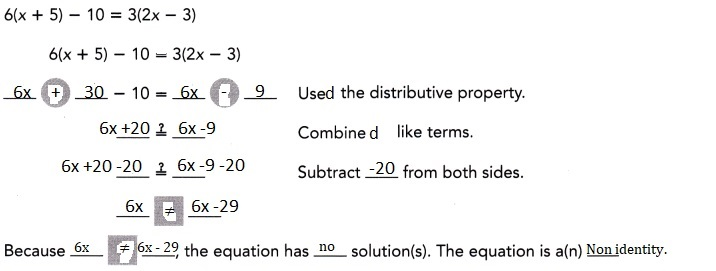Try substituting some values of x into the equation. If you find that the left side is always equal to the right side, the equation is an identity.### Math in Focus Course 3A Practice 3.2 Answer Key

Tell whether each equation has one solution, no solution, or an infinite number of solutions. Justify your answer.

Question 1.
2x – 3 = -2$$\left(\frac{3}{2}-x\right)$$
Equation has one solution,

Explanation: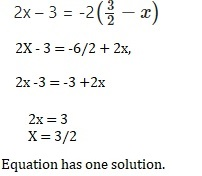Question 2.
2x + 5 = -4$$\left(\frac{3}{2}-x\right)$$
Equation has one solution,

Explanation: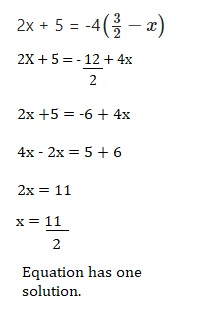Question 3.
3x + 5 = 2x – 7
x = -12, Equation has one solution,

Explanation:
Given 3x + 5 = 2x -7,
3x – 2x = -7 -5,
x = -12,
Equation has one solution.

Question 4.
5y + (86 – y) = 86 + 4y
The equation is identity and
an identity equation has infinetly many solutions,

Explanation:
Given 5y + (86 – y) = 86 + 4y upon solving
5y +86 -y = 86 + 4y,
4y + 86 = 86 + 4y ,the equation is identity and
an identity equation has infinetly many solutions.

Question 5.
0.5(6x – 3) = 3(1 + x)
No solution,

Explanation:
Given 0.5(6x -3) = 3(1 + x),
(0.5 X  6x) – (0.5 X 3) = 3 +3x,
3x -1.5 = 3 + 3x
-1.5 ≠ 3, So the equation has no solution,

Question 6.
4(18a — 7) + 40 = 3(4 + 24a)
The equation is identity and
an identity equation has infinetly many solutions,

Explanation:
4 X 18a – 4 X 7 + 40 = 12 + 72a
72 a – 28 +40 = 72a+ 12
72a + 12 = 72a+12,
both are equal so infinite solutions.

Question 7.
$$\frac{1}{7}$$(7x – 21) = 8x + 7x – 24
Equation has one solution,

Explanation:
Given $$\frac{1}{7}$$(7x – 21) = 8x + 7x – 24,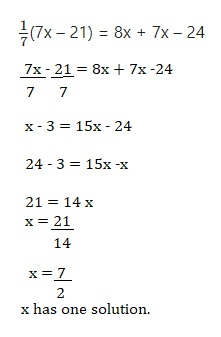Question 8.
$$\frac{1}{6}$$(12x – 18) = 2$$\left(x-\frac{3}{2}\right)$$
The equation is identity and
an identity equation has infinetly many solutions,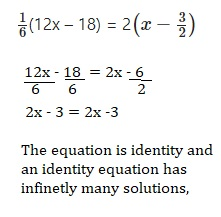Question 9.
7 – 0.75x = -7$$\left(\frac{3}{28} x+1\right)$$
No solution,

Explanation: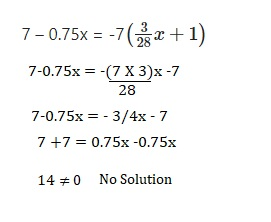Question 10.
6 + 0.5y = -2$$\left(3-\frac{1}{4} y\right)$$
No solution,

Explanation: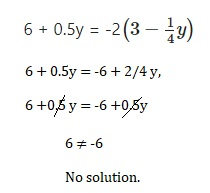Question 11.
$$\frac{x-3}{4}$$ = 0.25x – 0.75
The equation is identity and
an identity equation has infinetly many solutions,

Explanation: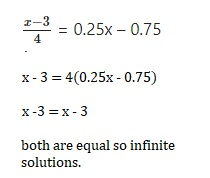Question 12.
$$\frac{1}{3}$$x + 5 = $$\frac{1}{6}$$(2x – 5)
No solution,

Explanation: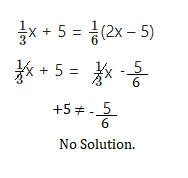Question 13.
Cabinet A is 5 inches taller than Cabinet B. Cabinet C is 3 inches taller than Cabinet B whose height is x inches.

a) Write algebraic expressions for the heights of cabinets A and C.
Cabinet A = 5 + x,
Cabinet C = 3 + x,

Explanation:
Given Cabinet B height is x inches as Cabinet A is 5 inches taller than Cabinet B
Cabinet A = 5 + x and Cabinet C is 3 inches taller than Cabinet B so Cabinet C = 3 + x.

b) If the total height of the three cabinets is (3x + 8) inches, can you solve for the height of Cabinet B? Explain.
We cannot solve for the height of Cabinet B
we need numbers.

Explanation:
Given the total height of the three cabinets is (3x + 8) inches,
we have Cabinet A = 5 + x, Cabinet B = x and Cabinet C = 3 + x,
5 + x + x +3 + x = 3x +8, We cannot solve for the height of Cabinet B
we need numbers.

Question 14.
A room’s floor is y meters long. Its width is 5 meters shorter.
If the perimeter of the floor is (4y + 1) meters, can you solve for its length? Explain.
There is not answer. Or, the problem needs more definition,

Explanation:
Room Floor Length(L) = y m.Width(W) y – 5 m.
Perimeter = 4y + 1,Find: Length(L),
Using perimeter formula P = 2L + 2W to get an equation to solve.
P = 4y + 1 = 2(y) + 2(y – 5),
4y + 1 = 2y + 2y – 10 => 1 = -10,
There is not answer. Or, the problem needs more definition.

Question 15.
Math Journal
Grace gave her sister a riddle: I have a number x. I add 15 to twice x to get result A.
I subtract 4 from x to get result B. I multiply result B by 3 to get result C.
Result A is equal to result C. Grace’s sister said the riddle cannot be solved,
but Grace thought otherwise. Who is right? Explain.
Grace is right she solved the riddle,

Explanation:
Given Grace gave her sister a riddle: I have a number x. I add 15 to twice x to get result A.
A= 15 + 2x, I subtract 4 from x to get result B. B = x -4,
I multiply result B by 3 to get result C. 3B = C ,
Result A is equal to result C, 15 + 2x = C = 3(x-4)
15 + 2x = 3x – 12,
15 + 12 = 3x -2x,
27 = x.
Grace is right she solved the riddle.

Question 16.

Math Journal Look at this “proof” that 2 = 0.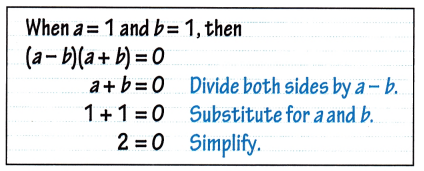What is wrong with this proof? How can a true statement lead to an inconsistent equation?